# 22.3: Spectral Properties of Arenes

$$\newcommand{\vecs}{\overset { \rightharpoonup} {\mathbf{#1}} }$$ $$\newcommand{\vecd}{\overset{-\!-\!\rightharpoonup}{\vphantom{a}\smash {#1}}}$$$$\newcommand{\id}{\mathrm{id}}$$ $$\newcommand{\Span}{\mathrm{span}}$$ $$\newcommand{\kernel}{\mathrm{null}\,}$$ $$\newcommand{\range}{\mathrm{range}\,}$$ $$\newcommand{\RealPart}{\mathrm{Re}}$$ $$\newcommand{\ImaginaryPart}{\mathrm{Im}}$$ $$\newcommand{\Argument}{\mathrm{Arg}}$$ $$\newcommand{\norm}{\| #1 \|}$$ $$\newcommand{\inner}{\langle #1, #2 \rangle}$$ $$\newcommand{\Span}{\mathrm{span}}$$ $$\newcommand{\id}{\mathrm{id}}$$ $$\newcommand{\Span}{\mathrm{span}}$$ $$\newcommand{\kernel}{\mathrm{null}\,}$$ $$\newcommand{\range}{\mathrm{range}\,}$$ $$\newcommand{\RealPart}{\mathrm{Re}}$$ $$\newcommand{\ImaginaryPart}{\mathrm{Im}}$$ $$\newcommand{\Argument}{\mathrm{Arg}}$$ $$\newcommand{\norm}{\| #1 \|}$$ $$\newcommand{\inner}{\langle #1, #2 \rangle}$$ $$\newcommand{\Span}{\mathrm{span}}$$$$\newcommand{\AA}{\unicode[.8,0]{x212B}}$$

## Infrared Spectra

The presence of a phenyl group in a compound can be ascertained with a fair degree of certainty from its infrared spectrum. For example, in Figure 22-1 we see the infrared spectra of methylbenzene, and of 1,2-, 1,3-, and 1,4-dimethylbenzene. That each spectrum is of a benzene derivative is apparent from certain common features. The two bands near $$1600 \: \text{cm}^{-1}$$ and $$1500 \: \text{cm}^{-1}$$, although of variable intensity, have been correlated with the stretching vibrations of the carbon-carbon bonds of the aromatic ring; also, the sharp bands near $$3030 \: \text{cm}^{-1}$$ are characteristic of aromatic $$\ce{C-H}$$ bonds. Other bands in the spectra, especially those between $$1650 \: \text{cm}^{-1}$$ and $$2000 \: \text{cm}^{-1}$$, between $$1225 \: \text{cm}^{-1}$$ and $$950 \: \text{cm}^{-1}$$, and below $$900 \: \text{cm}^{-1}$$, have been correlated with the number and position of ring substituents. Although we shall not document all these various bands in detail, each of the spectra in Figure 22-1 is marked to show some of the correlations that have been made.Figure 22-1: Infrared spectra of methylbenzene and the 1,2-, 1,3-, and 1,4-dimethylbenzenes. The number and positions of ring substituents determine the pattern of the low-intensity bands in the region $$2000 \: \text{cm}^{-1}$$ to $$1650 \: \text{cm}^{-1}$$ and the positions of the stronger bands in the region $$800 \: \text{cm}^{-1}$$ to $$690 \: \text{cm}^{-1}$$. The sharp bands near $$3030 \: \text{cm}^{-1}$$ arise from $$\ce{C-H}$$ stretching vibrations.

## Electronic Absorption Spectra

Compared to straight-chain conjugated polyenes, aromatic compounds have relatively complex absorption spectra with several bands in the ultraviolet region. Benzene and the alkylbenzenes show two bands in which we shall be primarily interested, one near $$200 \: \text{nm}$$ and the other near $$260 \: \text{nm}$$. The $$200$$-$$\text{nm}$$ band is of fairly high intensity and corresponds to excitation of a $$\pi$$ electron of the conjugated system to a $$\pi^*$$ orbital (i.e., a $$\pi \rightarrow \pi^8*$$ transition). The excited state has significant contributions from dipolar structures such as $$1$$: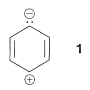This is analogous to the absorption bands of conjugated dienes (Section 9-9B) except that the wavelength of absorption of benzenes is shorter. In fact, the $$200$$-$$\text{nm}$$ absorptions of benzene and the alkylbenzenes are just beyond the range of most commercial quartz spectrometers. However, these absorptions (which we say arise from the benzene chromophore$$^2$$) are intensified and shifted to longer wavelengths when the conjugated system is extended by replacement of the ring hydrogens by unsaturated groups (e.g., $$\ce{-CH=CH_2}$$, $$\ce{-C \equiv CH}$$, $$\ce{-CH=O}$$, and $$\ce{-C \equiv N}$$; see Table 22-2). The delocalized $$\pi$$-electron system of the absorbing chromophore now includes the electrons of the unsaturated substituent as well as those of the ring. In the specific case of ethenylbenzene the excited state is a hybrid structure composite of $$2a$$ and $$2b$$ and other related dipolar structures:Table 22-2: Effect of Conjugation on Electronic Absorption by the Benzene ChromophoreSimilar effects are observed for benzene derivatives in which the substituent has unshared electron pairs that can be conjugated with the benzene ring (e.g., $$\ce{-NH_2}$$, $$\ce{-OH}$$, $$\ce{-Cl}$$). An unshared electron pair is to some extent delocalized to become a part of the aromatic $$\pi$$-electron system in both the ground and excited states, but more importantly in the excited state. This is illustrated for benzenamine (aniline) by the following structures, which contribute to the hybrid structure:The data of Table 22-3 show the effect on the benzene chromophore of this type of substituent - the substituent often being called an auxochrome.$$^2$$ This term means that, although the substituent itself is not responsible for the absorption band, it shifts the absorption of the chromophoric group, in this case the benzene ring, toward longer wavelengths. The auxochromic groups usually increase the intensity of the absorption also.

Table 22-3: Effect of Auxochromic Substituents on Electronic Absorption by the Benzene ChromophoreFigure 22-3, is of relatively low intensity and is found under high resolution to be a composite of several narrow peaks. It appears to be characteristic of aromatic hydrocarbons because no analogous band is found in the spectra of conjugated acyclic polyenes. For this reason it often is called the benzenoid band. The position and intensity of this band, like the one at shorter wavelengths, is affected by the nature of the ring substituents, particularly by those that extend the conjugated system, as may be seen from the date in Table 22-4.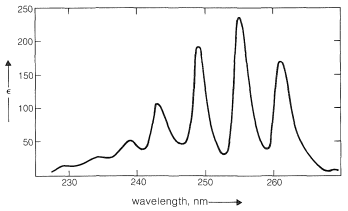Figure 22-3: Ultraviolet absorption spectrum of benzene (in cyclohexane) showing the "benzenoid" band.

Table 22-4: Effects of Structure on Electronic Absorption Corresponding to the Benzenoid BandThe benzenoid band corresponds to a low-energy $$\pi \rightarrow \pi^*$$ transition of the benzene molecules. The absorption intensity is weak because the $$\pi^*$$ state involved has the same electronic symmetry as the ground state of benzene, and transitions between symmetrical states usually are forbidden. The transitions are observed in this case only because the vibrations of the ring cause it to be slightly distorted at given instants. In the valence-bond treatment this excited state of benzene is an antibonding state of the $$\pi$$ electrons.

The electronic spectra of polynuclear aromatic hydrocarbons such as naphthalene and anthracene, in which aromatic rings are fused together in a linear manner, resemble the spectrum of benzene except that the bands are shifted to longer wavelengths. In fact, with the four linearly connected rings of naphthacene, the benzenoid band is shifted far enough to longer wavelengths to be in the visible region of the spectrum (see Table 22-4). Accordingly, naphthacene is yellow. The next higher member, pentacene, is blue.

Compounds such as phenanthrene, chrysene, and pyrene, in which the aromatic rings are fused in an angular manner, have complex electronic spectra with considerable fine structure. The $$\lambda_\text{max}$$ values normally are at shorter wavelengths than those of their linear isomers.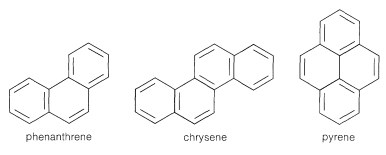## Nuclear Magnetic Resonance Spectra

The chemical shifts of arene protons ($$6.5 \: \text{ppm}$$ to $$8.0 \: \text{ppm}$$) characteristically are toward lower magnetic fields than those of protons attached to ordinary double bonds ($$4.6 \: \text{ppm}$$ to $$6.9 \: \text{ppm}$$). The difference of about $$2 \: \text{ppm}$$ cannot be easily explained because the hydrogens in both types of systems are bonded to carbon through $$sp^2$$-$$\sigma$$ bonds (Sections 6-4C and 6-5A).

At least part of the chemical-shift difference between arene protons and alkene protons is the result of the special property of $$\pi$$ electrons in aromatic systems of circulating freely above and below the plane of the carbon nuclei, as shown in Figure 22-4. When a molecule such as benzene is subjected to a magnetic field that has a component perpendicular to the plane of the ring, the electrons circulate around the ring in such a way as to produce a local magnetic dipole in the direction opposite to the applied field. This diamagnetic shielding effect acts to reduce the applied field in the center of the ring. Therefore, if a proton could be located in the center of the ring, the applied field would have to be higher than normal to counteract the local diamagnetic field and bring the proton into resonance. A proton outside the ring is affected in the opposite way (paramagnetic deshielding effect) because, as can be seen from the diagram, such protons are located in the return path of the lines of force associated with the local field and thus are in a field greater than that arising from the external magnet alone. When the plane of the molecule is oriented parallel to the field, the diamagnetic circulation is cut off. As a result, as the molecules tumble over and over in the liquid the component of magnetization perpendicular to the plane of the ring varies rapidly. Nonetheless, a substantial net paramagnetic effect is experienced by the ring hydrogens. The resonance line positions therefore are shifted to lower magnetic fields.Figure 9-26. The strength of the induced magnetic field, or dipole, is proportional to the applied field.

Strong evidence in confirmation of the above explanation of the chemical shifts of aromatic hydrogens is provided by a study of the cyclic conjugated polyene annulene, which has hydrogens both "inside" and "outside" the ring: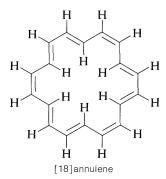The inside hydrogens are strongly deshielded, coming at $$1.9 \: \text{ppm}$$ upfield from tetramethylsilane, while the outside hydrogens are deshielded and come at $$8.8 \: \text{ppm}$$ downfield from TMS. As we shall see, the ring current effect is quite general and constitutes a widely used test for aromatic character in conjugated polyene ring systems.

In general, the spin-spin splittings observed between the five protons of a phenyl group can be extremely complex. An example is afforded by nitrobenzene (Figure 22-5), which has different chemical shifts for its ortho, meta, and para hydrogens and six different spin-spin interaction constants: $$J_{23}$$, $$J_{24}$$, $$J_{25}$$, $$J_{26}$$, $$J_{34}$$, $$J_{35}$$, (the subscripts correspond to position numbers of the protons):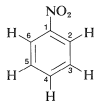Figure 22-5: Nmr spectrum of nitrobenzene at $$60 \: \text{MHz}$$ with reference to TMS at $$0.00 \: \text{ppm}$$.

Such a spectrum is much too complex to be analyzed by any simple procedure. Nonetheless, nuclear magnetic resonance can be useful in assigning structures to aromatic derivatives, particularly in conjunction with integrated line intensities and approximate values of the coupling constants between the ring hydrogens, as shown below:$$^2$$A chromophore is a grouping of atoms in an organic molecule that gives rise to color, or has the potential of doing so when other groups called auxochromes are present (also see Section 28-4).